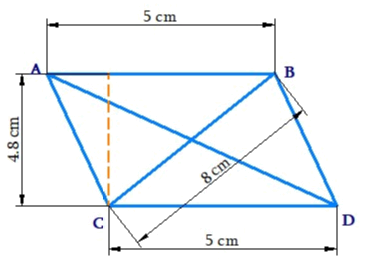# Ex.11.2 Q6 Mensuration Solution - NCERT Maths Class 8

Go back to  'Ex.11.2'

## Question

Find the area of a rhombus whose side is $$5\,\rm{ cm}$$ and whose altitude is $$4.8\,\rm{ cm.}$$ If one of its diagonals is $$8 \,\rm{cm}$$ long, find the length of the other diagonal.

Video Solution
Mensuration
Ex 11.2 | Question 6

## Text Solution

What is Known?

One of the diagonal, side and attitude of the rhombus.

What is unknown?

Area of the rhombus and length of the diagonal.

Reasoning:

Rhombus is a special case of parallelogram and the area of parallelogram is product of its base and height.

Steps:Let the length of the other diagonal of rhombus is $$x.$$

Area of the rhombus $$ABCD$$

\begin{align} &= {\text{Base}} \times {\text{Length}}\\& = 5\,{\rm{cm}} \times 4.8 \, \rm{cm}\\ &= 24.0\,{{\rm{cm}}^2}\end{align}

Also,

Area of rhombus $$=\frac{1}{2}\times$$ Product of its diagonals

\begin{align} 24\,\text{cm}^{2} &=\frac{1}{2}(AD\times CB) \\ 24\,\text{cm}^{2}&=\frac{1}{2}(x\times 8\,\text{cm}) \\ x\times 4\,\text{cm}&=24\,\text{c}{{\text{m}}^{2}} \\ x&=6\,\text{cm}\end{align}

Thus, area of the rhombus is $$24.0\,{{\rm{m}}^2}$$ and length of the diagonals is $$6\,{\rm{cm}}$$ .

Learn from the best math teachers and top your exams

• Live one on one classroom and doubt clearing
• Practice worksheets in and after class for conceptual clarity
• Personalized curriculum to keep up with school